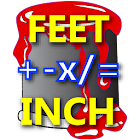Feet Inch Material Calculator

All Android applications categories

All Android games categories# Feet Inch Material Calculator

by: 21 9.4

9.4 Users
rating

## Screenshots

Description

- It's the most completed feet inch calculator plus common construction material calculation.
- Standard calculator look and feel to calculate Length, Area, and Volume by enter feet, inch and fraction.
- Input can be feet & inch (e.g. 2'6 1/2"), just inch (e.g. 5 3/4"), decimal feet (e.g 3.75 ft), or decimal inch (e.g 2.25") in the same calculation.
- Convert values between different units with the dimension.
- Convert feet and inches between decimal value and feet inch fraction format.
- Supported units: Feet, Inch, Yards, Meter, CM, MM, and Board Foot.
- It's easy to calculate lumber's linear board foot usage by enter the lumber's width, length, depth, and quantity, then convert to Board Foot unit.
- Function keys: Square, Square Root, Percent, sin, cos, tan, asin, acos, atan, Pythagorean Theorem and PI keys.
- Angle can be entered as Degree or Radian.
- With Percent key, it's easy to calculate the material waste.
- With Pythagorean Theorem key, just need two clicks to calculate hypotenuse of Right Triangle; no more need use with Square or Square Root keys.
- With the sin, cos, and tan keys, you can calculate distance with angle.
- Support M+ and M- memory keys to recall saved values.
- Calculate the Materials needed base on Length, Area, and Volume.
- User can customize the materials coverage to calculate the same category materials base on the needed.
- Supported material calculation: Concrete, Fence Post and boards, Gravel, Paint, Plywood, Roof Shingles, and Wall Studs.
- Built-in on-line user guide (Internet access required).
- Provide Haptic feedback (can turn off).
- Paperless tape feature.

User can request on-line user guide from the developer before purchase the app.

User may send additional material calculation request to the developer by providing the calculation logic, developer will review and may add into the app in next update.

1.00.102:
Add sin, cos, and tan keys under shifted mode.
Angle value can be entered as degree or radian.
Add configured setting to not shown FT or IN unit at the end when the value displays in Feet Inch format (per user requested).

Tags: fabric calcalation , convert cm to fractional inches , feet inch material , code calculator cos sin tag html , code about calculator add sin cos tag , 1 feet inch , calculator feetinch , الة حاسبة inch , calculatie mobiel feet yard

from 21 reviews

"Awesome"

9.4